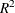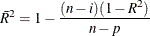# The GLMSELECT Procedure

### Displayed Output

Subsections:

The following sections describe the displayed output produced by PROC GLMSELECT. The output is organized into various tables, which are discussed in order of appearance. Note that the contents of a table might change depending on the options that you specify.

#### Model Information

The "Model Information" table displays basic information about the data sets and the settings used to control effect selection. These settings include the following:

• the selection method

• the criteria used to select effects, stop the selection, and choose the selected model

• the effect hierarchy enforced

The ODS name of the "Model Information" table is ModelInfo.

#### Performance Settings

The "Performance Settings" table displays settings that affect performance. These settings include whether threading is enabled and the number of CPUs available as well as the method used to build the crossproduct matrices. This table is displayed only if you specify the DETAILS option in the PERFORMANCE statement. The ODS name of the "Performance Settings" table is PerfSettings.

#### Number of Observations

The "Number of Observations" table displays the number of observations read from the input data set and the number of observations used in the analysis. If you specify a FREQ statement, the table also displays the sum of frequencies read and used. If you use a PARTITION statement, the table also displays the number of observations used for each data role. If you specify TESTDATA= or VALDATA= data sets in the PROC GLMSELECT statement, then "Number of Observations" tables are also produced for these data sets. The ODS name of the "Number of Observations" table is NObs.

#### Class Level Information

The "Class Level Information" table lists the levels of every variable specified in the CLASS statement. The ODS name of the "Class Level Information" table is ClassLevelInfo.

#### Class Level Coding

The "Class Level Coding" table shows the coding used for variables specified in the CLASS statement. The ODS name of the "Class Level Coding" table is ClassLevelCoding.

#### Dimensions

The "Dimensions" table displays information about the number of effects and the number of parameters from which the selected model is chosen. If you use split classification variables, then this table also includes the number of effects after splitting is taken into account. The ODS name of the "Dimensions" table is Dimensions.

#### Candidates

The "Candidates" table displays the effect names and values of the criterion used to select entering or departing effects at each step of the selection process. The effects are displayed in sorted order from best to worst of the selection criterion. You request this table with the DETAILS= option in the MODEL statement. The ODS name of the "Candidates" table is Candidates.

#### Selection Summary

The "Selection Summary" table displays details about the sequence of steps of the selection process. For each step, the effect that was entered or dropped is displayed along with the statistics used to select the effect, stop the selection, and choose the selected model. You can request that additional statistics be displayed with the STATS= option in the MODEL statement. For all criteria that you can use for model selection, the steps at which the optimal values of these criteria occur are also indicated. The ODS name of the "Selection Summary" table is SelectionSummary.

#### Stop Reason

The "Stop Reason" table displays the reason why the selection stopped. To facilitate programmatic use of this table, an integer code is assigned to each reason and is included if you output this table by using an ODS OUTPUT statement. The reasons and their associated codes follow:

Code

Stop Reason

1

maximum number of steps done

2

specified number of steps done

3

specified number of effects in model

4

stopping criterion at local optimum

5

model is an exact fit

6

all entering effects are linearly dependent on those in the model

7

all effects are in the model

8

all effects have been dropped

9

requested full least squares fit completed

10

stepwise selection is cycling

11

dropping any effect does not improve the selection criterion

12

no effects are significant at the specified SLE or SLS levels

13

adding or dropping any effect does not improve the selection criterion

14

all remaining effects are required

The ODS name of the "Stop Reason" table is StopReason.

#### Stop Details

The "Stop Details" table compares the optimal value of the stopping criterion at the final model with how it would change if the best candidate effect were to enter or leave the model. The ODS name of the "Stop Details" table is StopDetails.

#### Selected Effects

The "Selected Effects" table displays a string containing the list of effects in the selected model. The ODS name of the "Selected Effects" table is SelectedEffects.

#### ANOVA

The "ANOVA" table displays an analysis of variance for the selected model. This table includes the following:

• the Source of the variation, Model for the fitted regression, Error for the residual error, and C Total for the total variation after correcting for the mean. The Uncorrected Total Variation is produced when the NOINT option is used.

• the degrees of freedom (DF) associated with the source

• the Sum of Squares for the term

• the Mean Square, the sum of squares divided by the degrees of freedom

• the F Value for testing the hypothesis that all parameters are zero except for the intercept. This is formed by dividing the mean square for Model by the mean square for Error.

• the Prob>F, the probability of getting a greater F statistic than that observed if the hypothesis is true. Note that these p-values are displayed only if you specify the "SHOWPVALUES" option in the MODEL statement. These p-values are generally liberal because they are not adjusted for the fact that the terms in the model have been selected.

You can request "ANOVA" tables for the models at each step of the selection process with the DETAILS= option in the MODEL statement. The ODS name of the "ANOVA" table is ANOVA.

#### Fit Statistics

The "Fit Statistics" table displays fit statistics for the selected model. The statistics displayed include the following:

• Root MSE, an estimate of the standard deviation of the error term. It is calculated as the square root of the mean square error.

• Dep Mean, the sample mean of the dependent variable

• R-square, a measure between 0 and 1 that indicates the portion of the (corrected) total variation attributed to the fit rather than left to residual error. It is calculated as SS(Model) divided by SS(Total). It is also called the coefficient of determination. It is the square of the multiple correlation—in other words, the square of the correlation between the dependent variable and the predicted values.

• Adj R-Sq, the adjusted, a version ofthat has been adjusted for degrees of freedom. It is calculated aswhere i is equal to 1 if there is an intercept and 0 otherwise, n is the number of observations used to fit the model, and p is the number of parameters in the model.

• fit criteria AIC, AICC, BIC, CP, and PRESS if they are used in the selection process or are requested with the STATS= option. See the section Criteria Used in Model Selection Methods for details and Table 48.10 for the formulas for evaluating these criteria.

• the CVPRESS statistic when cross validation is used in the selection process. See the section Cross Validation for details.

• the average square errors (ASE) on the training, validation, and test data. See the section Using Validation and Test Data for details.

You can request "Fit Statistics" tables for the models at each step of the selection process with the DETAILS= option in the MODEL statement. The ODS name of the "Fit Statistics" table is FitStatistics.

#### Cross Validation Details

The "Cross Validation Details" table displays the following:

• the fold number

• the number of observations used for fitting

• the number of observations omitted

• the predicted residual sum of squares on the omitted observations

You can request this table with the CVDETAILS= option in the MODEL statement whenever cross validation is used in the selection process. This table is displayed for the selected model, but you can request this table at each step of the selection process by using the DETAILS= option in the MODEL statement. The ODS name of the "Cross Validation Details" table is CVDetails.

#### Parameter Estimates

The "Parameter Estimates" table displays the parameters in the selected model and their estimates. The information displayed for each parameter in the selected model includes the following:

• the parameter label that includes the effect name and level information for effects containing classification variables

• the degrees of freedom (DF) for the parameter. There is one degree of freedom unless the model is not full rank.

• the parameter estimate

• the standard error, which is the estimate of the standard deviation of the parameter estimate

• T for H0: Parameter=0, the t test that the parameter is zero. This is computed as the parameter estimate divided by the standard error.

• the Prob > |T|, the probability that a t statistic would obtain a greater absolute value than that observed given that the true parameter is zero. This is the two-tailed significance probability. Note that these p-values are displayed only if you specify the SHOWPVALUES option in the MODEL statement. These p-values are generally liberal because they are not adjusted for the fact that the terms in the model have been selected.

If cross validation is used in the selection process, then you can request that estimates of the parameters for each cross validation fold be included in the "Parameter Estimates" table by using the CVDETAILS= option in the MODEL statement. You can request "Parameter Estimates" tables for the models at each step of the selection process with the DETAILS= option in the MODEL statement. The ODS name of the "Parameter Estimates" table is ParameterEstimates.

#### Score Information

For each SCORE statement, the "Score Information" table displays the names of the score input and output data sets, and the number of observations that were read and successfully scored. The ODS name of the "Score Information" table is ScoreInfo.

#### Timing Breakdown

The "Timing Breakdown" table displays a broad breakdown of where time was spent in the PROC GLMSELECT step. This table is displayed only if you specify the DETAILS option in the PERFORMANCE statement. The ODS name of the "Timing Breakdown" table is Timing.